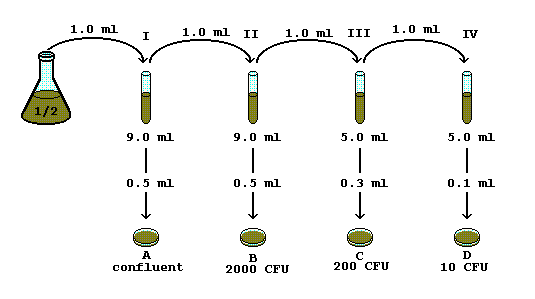# Serial Dilution Problem # 11. Which is the countable plate? ______ # CFU = ______
2. What is the SDF? ______
3. Calculate the ITDF for each tube:
• I
• II
• III
• IV

4. Calculate the TSDF for the countable plate.

5. Calculate the PDF for the countable plate.

6. Calculate the FDF for the countable plate.

7. Calculate the CFU/ml for the countable plate.

Thanks to Tim Allen for providing this problem set.